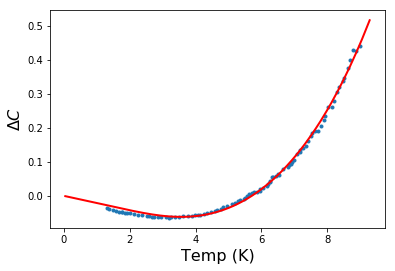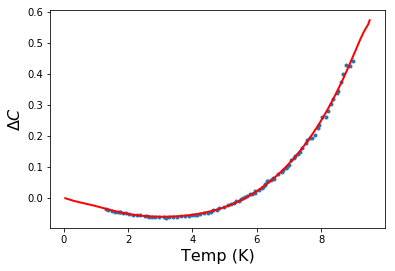*

## The Alpha Model

#### How to find the superconducting energy gap from specific heat measurements

It is possible to estimate the superconducting energy gap $\Delta$ by fitting specific heat data in the superconducting state using the Alpha Model, a semi empirical model loosely based on BCS theory, and created by Padamsee et al 1. Our most recent fits have been done using the more recent version by Johnston 2, which allows direct integration of the specific heat data to find the size of the gap. In this model the ratio $\alpha = \Delta/k_BT_c$, is a free parameter rather than fixing $\alpha$ at the BCS value of 1.764, although the gap in the model has the same temperature dependence as the BCS model. The calculation is based on the universal expression of the entropy, $S = k_B\sum f ln(f)$, where $f$ is the Fermi distribution function. The sum can be turned into an integral over the energy range of the quasiparticles, and for a given $\alpha, T_c$, and $\gamma$, the entropy is calculated as a function of temperature. The calculated specific heat can then be found by taking the derivative of the entropy with respect to the temperature. In the Johnston version the derivative of the entropy integral is done analytically and a new integral results that can be used to find the specific heat directly.

One of the additional complications of fitting the specific heat to the Alpha Model is that the fit is sensitive to symmetry of the superconducting order parameter. The original versions of both Padamsee and Johnston use s-wave pairing. We modified the integral expressions for d-wave symmetry. In most cases we fit the data using both forms of the equation, and pick the best fit to determine $\alpha$, and ideally determine the pairing symmetry, aAlpha Model fit of $\kappa$-(BEDT-TTF)$_2$Cu(NCS)$_2$ using s-wave pairing symmetry. Data is from Carrington. 3Alpha Model fit of $\kappa$-(BEDT-TTF)$_2$Cu(NCS)$_2$ using d-wave pairing symmetry. Notice that the d-wave fit is better than the s-wave fit. question which is still not completely settled in the organics. We find d-wave as the best fit for $\kappa$ET-CuNCS as did Taylor et al. 3 although we find s-wave as the best fit for the other organics, which is contrary to some of the other evidence in, for example, the $\kappa$ET-Br material 4. Part of the challenge in organics is that the lattice is soft, so the phonon contribution to the specific heat continues to low temperatures. One way to subtract the phonon contribution is to measure the specific heat at a magnetic field large enough to quench the superconductivity and subtract that curve from the specific heat measured with no magnetic field. Wth this method the phonon contribution is subtracted, but the electronic linear term is also subtracted and mixed with the phonon terms. The linear term determines the constant $\gamma$, which is a measure of the electronic density of states. The ability to determine $\gamma$ is critical to getting a good result for the $\alpha$ fits. It is unclear what our conclusion should be for the pairing symmetry, particularly if we believe that all the organics have the same pairing. We have also noticed that the shape of the fits are not perfect with s or d-wave pairing, which suggests the possibly that the pairing has some other symmetry such as s+d, or anisotropic s-wave, a notion supported by some experiments 5 and recent theory 6.
Material $\alpha$ $g^*/g$ $T_c$(K) $H_p$(T) $\alpha_M$ $H_{orb}^0$(T) $\xi_{\bot}$(Å) $H_{c2}^0\bot$(T) $\xi_{||}$(Å) $\ell$(Å) r = $\ell/\xi$
$\kappa$-(BEDT-TTF)$_2$Cu(NCS)$_2$ 3.0 7 1.26 8 9.6 21.6 4.9 130 9 13 6.0 74 900 10 12
$\kappa$-(BEDT-TTF)$_2$Cu(NCS)$_2$ 1.75 >3.5 0 1.5 4.5 4.9 5.5 140 0.28 343 600 1.7
$\beta$"-(BEDT-TTF)$_2$SF$_5$CH$_2$CF$_2$SO$_3$ 1.94 11 1.0 8 4.5 9.2 3.9 75 9 21 1.6 23, 30 122 520 3.6
$\alpha$-(ET)$_2$NH$_4$Hg(SCN)$_4$ 1.76 12 0.86 8 0.96 2.1 5.5 8.1 13 53 0.14 13 485 681 13 1.4
$\lambda$-(BETS)$_2$GaCl$_4$ 1.83 14 1.0 8 4.3 8.3 3.9 23.115 31.5 2.9 107 170 16 1.6
$\kappa$-(ET)$_2$Cu[N(CN)$_2$]Br 2.77 7 1.4 8 11.5 23.8 9.6 161 17 12 11 55 260 18 4.7
CeCoIn$_5$ || 3.0319, 20 0.7319 2.16 9.44 6.5 43.5 21 23 2 128 810 6.3
CeCoIn5 $\bot$ 1.0 1 2.16 4.8 3.7 17 44 17 44 1500 34
KFe$_2$As$_2$ 1.75 22 1.3 23 3.14 4.84 2.9 9.9 48 2.0 128 177024 14
LiFeAs 1.89 25 1.0 17.6 34.9 2.1 51 26 21 30 33 5500 26 167
(TMTSF)$_2$ClO$_4$ 1.519, 20 219 1.1 1.5 2.5 6 21 10 2 1000 810 1.2
Table 1. Note that || and $\bot$ values may be different. These parameters will help determine which materials are good candidates for a first-order $H_{c2}$ transition or the FFLO state. $H_{c2}^0\bot$(T) is the extrapolated value from the slope near $T_c$.27 For $\kappa$-(BEDT-TTF)$_2$Cu(NCS)$_2$ or other Pauli limited superconductors the slope near $T_c$ may not related to the coherence length and $H_{c2||}$ is estimated from $H_{c2}^0\bot$(T) and the anisotropy of the London penetration depth.28 To be consistent, we always compare $\ell$ and $\xi$ in the parallel direction (in the conducting planes) to calculate r. The mean free path for $\kappa$-(BEDT-TTF)$_2$Cu(NCS)$_2$ under pressure is estimated.

The table above shows the values of $\alpha$ from our fits of specific heat measurements. We have collected other parameters, in particular the Maki parameter $\alpha_M$ (see the Intro to FFLO page) all of which are predictors for inhomogeneous superconductivity.

Material $T_c$(K) $\lambda_{||} (\mu$m) $\lambda_{\bot}(\mu$m) $E_F$(meV) $m^*$ $V_F$(m/s) $\Delta$(meV) $\ell$(Å) r = $\ell/\xi$
$\kappa$-(BEDT-TTF)$_2$Cu(NCS)$_2$ 9.6 1.2 100 9.6 9 3.5 4.45e4 2.5 900 10 13
$\kappa$-(BEDT-TTF)$_2$Cu(NCS)$_2$ 1.75 1.5 ?? ?? 9.6 2.5 4.45e4 ?? 600 1.7
$\beta$"-(BEDT-TTF)$_2$SF$_5$CH$_2$CF$_2$SO$_3$ 4.5 ?? ?? 11.5 9 2.0 4.49e4 23, 30 0.75 520 3.6
$\alpha$-(ET)$_2$NH$_4$Hg(SCN)$_4$ 0.96 ?? ?? 26.1 13 2.5 6.06e4 13 0.15 681 13 1.4
$\lambda$-(BETS)$_2$GaCl$_4$ 4.3 5.0 20 20.915 3.6 4.52e4 0.66 170 16 1.6
$\kappa$-(ET)$_2$Cu[N(CN)$_2$]Br 11.5 1.8 100 20.1 17 3.0 4.85e4 2.7 260 18 4.7
CeCoIn$_5$ 2.16 0.19 0.28 43.5 21 23 2 0.56 810 6.3
KFe$_2$As$_2$ 4.84 2.5 2.5 9.9 48 2.0 0.73 177024 14
LiFeAs 17.6 2.5 2.5 51 26 21 30 2.9 5500 26 167
(TMTSF)$_2$ClO$_4$ 1.1 0.5 40 6 21 10 2 0.14 810 1.2
Table 2. $E_F$ and $m^*$ are derived from quantum oscillation experiments.

2224

1 Padamsee, H. Neighbor, J. Shiffman, C., "Quasiparticle phenomenology for thermodynamics of strong- coupling superconductors," J. Low Temp. Phys. , 12, 387 (1973).
2 Johnston, D.C. Elaboration of the α-model derived from the BCS theory of superconductivity. Superconduct. Sci. Technol. 26, 115011 (2013).
3 Taylor, O.J. Carrington, A. Schlueter, J.A., "Specific-Heat Measurements of the Gap Structure of the Organic Superconductors $\kappa$-(BEDT-TTF)$_2$Cu[N(CN)$_2$]Br and $\kappa$-(ET)$_2$Cu(NCS)$_2$," Phys. Rev. Lett. 99, 057001 (2007).
4 Nakazawa, Y. Kanoda, K., "Low-temperature specific heat of $\kappa$-(BEDT-TTF)$_2$Cu[N(CN)$_2$]Br in the superconducting state," Phys. Rev. B 55, R8670 (1997).
5 Analytis, J.G. Ardavan, A. Blundell, S.J. Owen, R.L. Garman, E.F. Jeynes, C. Powell, B.J. Effect of Irradiation-Induced Disorder on the Conductivity and Critical Temperature of the Organic Superconductor $\kappa$-(BEDT-TTF)$_2$Cu(NCS)$_2$," Phys. Rev. Lett. 96, 177002 (2006).
6 Zantout, K. Altmeyer, M. Backes, S. Valentí, R., "Superconductivity in correlated BEDT-TTF molecular conductors: Critical temperatures and gap symmetries," Phys. Rev. B 97, 014530 (2018).
7 Taylor, O.J. Carrington, A. Schlueter, J.A., "Specific-Heat Measurements of the Gap Structure of the Organic Superconductors $\kappa$-(BEDT-TTF)$_2$Cu[N(CN)$_2$]Br and $\kappa$-(ET)$_2$Cu(NCS)$_2$," Phys. Rev. Lett. 99, 057001 (2007).
8 McKenzie, R.H. Wilson’s ratio and the spin splitting of magnetic oscillations in quasi-two-dimensional metals. arXiv , arXiv.9905044 (1999).
9 Wosnitza, J. "FFLO States in Layered Organic Superconductors," Annalen der Physik 530, 1700282 (2018).
10 Mihut, I. Agosta, C. Martin, C. Mielke, C. Coffey, T., "Incoherent Bragg reflection and Fermi-surface hot spots in a quasi-two-dimensional metal," Phys. Rev. B 73, 125118 (2006).
11 Wanka, S. Hagel, J. Beckmann, D. Wosnitza, J. Schlueter, J.A. Williams, J.M. Nixon, P.G. Winter, R.W. Gard, G.L., "Specific heat and critical fields of the organic superconductor $\beta$"-(BEDT-TTF)$_2$SF$_5$CH$_2$CF$_2$SO$_3$," Phys. Rev. B 57, 3084 (1998).
12 Elsinger, H. Wosnitza, J. Wanka, S. Hagel, J. Schweitzer, D. Strunz, W., "$\kappa$-(BEDT-TTF)$_2$Cu[N(CN)$_2$]Br: A Fully Gapped Strong-Coupling Superconductor," Phys. Rev. Lett. 84, 6098 (2000).
13 Coffey, T. Martin, C. Agosta, C.C. Kinoshota, T. Tokumoto, M., "Bulk two-dimensional Pauli-limited superconductor," Phys. Rev. B 82, 212502 (2010).
14 Imajo, S. Kanda, N. Yamashita, S. Akutsu, H. Nakazawa, Y. Kumagai, H. Kobayashi, T. Kawamoto, A., "Thermodynamic Evidence of d-Wave Superconductivity of the Organic Superconductor $\lambda$-(BETS)$_2$GaCl$_4$," J. Phys. Soc. Jpn. 85, 043705 (2016).
15 Coniglio, W.A. Winter, L.E. Cho, K. Agosta, C.C. Fravel, B. Montgomery, L.K., "Superconducting phase diagram and FFLO signature in $\lambda$-(BETS)$_2$GaCl$_4$ from rf penetration depth measurements," Phys. Rev. B 83, 224507 (2011).
16 Mielke, C. Singleton, J. Nam, M.S. Harrison, N. Agosta, C.C. Fravel, B. Montgomery, L.K., "Superconducting properties and Fermi-surface topology of the quasi-two-dimensional organic superconductor λ-(BETS)2GaCl4 (BETS= bis(ethylene-dithio)tetraselenafulvalene)," J. Phys. Condens. Matter 13, 8325 (2001).
17 Kwok, W. Welp, U. Carlson, K. Crabtree, G. Vandervoort, K. Wang, H. Kini, A. Williams, J.Stupka, D. Montgomery, L., "Unusual behavior in the upper critical magnetic fields of the ambient-pressure organic superconductor $\kappa$-(BEDT-TTF)$_2$Cu[N(CN)$_2$]Br [where BEDT-TTF represents bis (ethylenedithio) tetrathiofulvalene]," Phys. Rev. B 42, 8686–8689 (1990).
18 Mielke, C. Harrison, N. Rickel, D. Lacerda, A. Vestal, R. Montgomery, L., "Fermi-surface topology of $\kappa$-(BEDT-TTF)$_2$Cu[N(CN)$_2$]Br at ambient pressure," Phys. Rev. B 56, 4309 (1997).
19 Petrovic, C. Pagliuso, P.G. Hundley, M.F. Movshovich, R. Sarrao, J.L. Thompson, J.D. Fisk, Z. Monthoux, P., "Heavy-fermion superconductivity in CeCoIn$_5$ at 2.3 K," J. Phys. Condens. Matter 13, L337 (2001).
20 Ikeda, S. Shishido, H. Nakashima, M. Settai, R. Aoki, D. Haga, Y. Harima, H. Aoki, Y. Namiki, T. Sato, H. et al., "Unconventional Superconductivity in CeCoIn$_5$ Studied by the Specific Heat and Magnetization Measurements," J. Phys. Soc. Jpn. 70, 2248 (2001).
21 Murphy, T.P. Hall, D. Palm, E.C. Tozer, S.W. Petrovic, C. Fisk, Z. Goodrich, R.G. Pagliuso, P.G. Sarrao, J.L. Thompson, J.D., "Anomalous superconductivity and field-induced magnetism in CeCoIn$_5$," Phys. Rev. B 65, (2002).
22 Abdel-Hafiez, M. Aswartham, S. Wurmehl, S. Grinenko, V. Hess, C. Drechsler, S.L. Johnston, S. Wolter, A.U.B. Büchner, B. Rosner, H. et al. Publisher’s Note: Specific heat and upper critical fields in KFe$_2$As$_2$ single crystals. Phys. Rev. B 85, 179901 (2012).
23 Hardy, F. Böhmer, A.E. Aoki, D. Burger, P. Wolf, T. Schweiss, P. Heid, R. Adelmann, P. Yao, Y.X. Kotliar, G. et al, "Evidence of Strong Correlations and Coherence-Incoherence Crossover in the Iron Pnictide Superconductor KFe$_2$As$_2$. Phys. Rev. Lett. 111, 027002 (2013).
24 Zocco, D.A. Grube, K. Eilers, F. Wolf, T. Löhneysen, H.V., "Pauli-Limited Multiband Superconductivity in KFe$_2$As$_2$," Phys. Rev. Lett. 111, 057007 (2013).
25 Kim, H. Tanatar, M.A. Song, Y.J. Kwon, Y.S. Prozorov, R., "Nodeless two-gap superconducting state in single crystals of the stoichiometric iron pnictide LiFeAs," Phys. Rev. B 83, 100502, (2011).
26 Cho, K. Kim, H. Tanatar, M. Song, Y. Kwon, Y. Coniglio, W. Agosta, C. Gurevich, A. Prozorov, R., "Anisotropic upper critical field and possible Fulde-Ferrel-Larkin-Ovchinnikov state in the stoichiometric pnictide superconductor LiFeAs," Phys. Rev. B 83, 060502 (2011).
27 Werthamer, N.R.; Helfand, E.; Hohenberg, P.C., "Temperature and Purity Dependence of the Superconducting Critical Field, $H_{c2}$. III. Electron Spin and Spin-Orbit Effects," Phys. Rev. 147, 295 (1966).
28 R. A. Klemm, A. Luther, and M. R. Beasley, "Theory of the upper critical field in layered superconductors," Phys. Rev. B 12, 877 (1975).
29 J. Muller, M. Lang, and F. Steglich, J. A. Schlueter, A. M. Kini, and U. Geiser, J. Mohtasham, R. W. Winter, and G. L. Gard, T. Sasaki, N. Toyota,”Comparative thermal-expansion study of $\beta$"-(BEDT-TTF)$_2$SF$_5$CH$_2$CF$_2$SO$_3$ and $\kappa$-(ET)$_2$Cu(NCS)$_2$: Uniaxial pressure coefficients of Tc and upper critical fields,” Phys. Rev. B 61, 11739 (2000).
30 K. Cho, B. E. Smith, W. A. Coniglio, L. E. Winter, C. C. Agosta, and J. A. Schlueter, “Upper critical field study in the organic superconductor β''- $\beta$"-(BEDT-TTF)$_2$SF$_5$CH$_2$CF$_2$SO$_3$: Possibility of the Fulde-Ferrell-Larkin-Ovchinnikov state,” Phys. Rev.B 79, 220507(R) (2009).
Last updated 15 Dec 2018
Webmaster
cagosta@clarku.edu